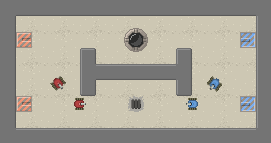# Customizing the Field¶

Game fields are based on a tilemap where each tile can only be occupied by a single object. This means they can be represented conveniently by an ASCII representation. You can instantiate fields from these ASCII representations as well. Suppose we create a file field.txt with the following contents:

```w w w w w w w w w w w w w w w w w
w _ _ _ _ _ _ _ _ _ _ _ _ _ _ _ w
w R _ _ _ _ _ _ C _ _ _ _ _ _ B w
w _ _ _ _ w _ _ _ _ _ w _ _ _ _ w
w _ _ _ _ w w w w w w w _ _ _ _ w
w _ _ _ _ w _ _ _ _ _ w _ _ _ _ w
w R _ _ _ _ _ _ A _ _ _ _ _ _ B w
w _ _ _ _ _ _ _ _ _ _ _ _ _ _ _ w
w w w w w w w w w w w w w w w w w
```

We can then load it up using the `domination.core.Field.from_string()` function, the map defined aboves looks like the image below:

```field = core.Field.from_string(open('field.txt').read())
core.Game(field=field).run()
```The default maps are randomly generated using the `FieldGenerator` class, it has a number of paramters for generating maps.

class `domination.core.``FieldGenerator`(width=41, height=24, tilesize=16, mirror=True, num_red=6, num_blue=6, num_points=3, num_ammo=6, num_crumbsource=0, wall_fill=0.4, wall_len=(3, 7), wall_width=4, wall_orientation=0.5, wall_gridsize=6)[source]

Generates field objects from random distribution

Create a FieldGenerator object with certain parameters for a random distribution of fields.

Parameters: width – The width of the field in tiles height – The height of the field in tiles tilesize – The size of each tile (don’t change from 16) mirror – Make a symmetrical map num_blue – The number of blue spawns num_red – The number of red spawns num_points – The number of controlpoints num_ammo – The number of ammo locations on the map num_crumbsource – The number of crumb fountains wall_fill – What portion of the map is occupied by walls wall_len – A range for the length of wall sections (min, max) wall_width – The width of each wall section wall_orientation – The probability that each wall will be placed horizontally i.e. that the walls length will be along a horizontal axis wall_gridsize – Place walls only at every n-th tile with their top-left
`generate`()[source]

Generates a new field using the parameters for random distribution set in the constructor.

Returns: A `Field` instance.